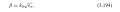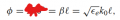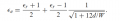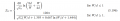# matching microstrip calculator with formula theory question

#### yef smith

Joined Aug 2, 2020
498
Hello, i have a microstrip operating at 10GHz L=4.91mm Er=9.6 d=0.254mm W=0.24mm as shown bellow.
from transmission line theory
Ee=0.5*(9.6+1)+0.5*(9.6-1)*(1/sqrt(1+12*(0.254/0.24)))=6.46mm
W/d=0.94
Z0=49.77 from formula (3.196)
k0=209.4[1/m]
I want to convert my 150 degrees into wavelength.
Is there a manual where i can find how many wavelength there are in the 150 degrees?
Thanks.#### ZCochran98

Joined Jul 24, 2018
261
1 wavelength = 2*pi radians = 360 degrees. So 150 degrees = 150/360 = 0.417 wavelengths

#### yef smith

Joined Aug 2, 2020
498
Hello ZCochran98,i want to measure my length using wavelenth how to do this in this case?
Thanks.

#### ZCochran98

Joined Jul 24, 2018
261
The electrical length of your microstrip (L=4.91mm) corresponds to, according to ADS' LineCalc tool, for your substrate, a 150-degree line, or 0.417 wavelengths (150degree/360 degree = 0.417*lambda).

Where this comes from is this: at 10 GHz, the wavelength of a signal is 30 mm in air (300*10^8 m/s/10 GHz -> 3/100 m or 30 mm [3cm]). However, in a substrate that is further reduced by a factor of $$\sqrt{\epsilon_r}$$, so the wavelength at 10 GHz in your substrate is 30 mm / sqrt(9.6) = 30/3.098, which is roughly 10 mm (9.68mm). So a 4.91 mm line is approximately 4.91/10 of a wavelength, or, precisely, 0.417 wavelengths.

Now, the estimate using $$\sqrt{\epsilon_r}$$ assumes the entire wave is inside the substrate. For a microstrip, some of the electric field is in the air, so you'd have to use the effective permittivity (which is probably closer to roughly 6.5ish based on a back-of-the-napkin estimate without looking at your W/d ratio - that's $$\epsilon_e$$ in your equations), which gives a wavelength of 11.8 mm, so, using that, 4.91 mm is about 0.416 of a wavelength (which is really close to ADS' predicted 0.417).

So, in other words, the degrees ADS gives you can be converted to fractions of a wavelength (electrical length/360 degrees), and wavelength can be found by $$\lambda = \frac{c}{f\sqrt{\epsilon_e}}$$.

Hope this helps!

•yef smith